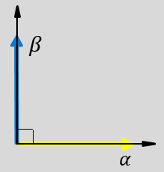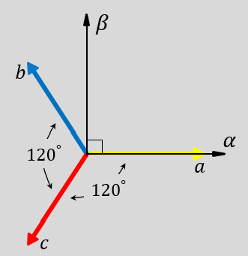Main Content

# Inverse Clarke Transform

Implement αβ to abc transformation

• Library:
• Motor Control Blockset / Controls / Math Transforms

•## Description

The Inverse Clarke Transform block computes the Inverse Clarke transformation of balanced, two-phase orthogonal components in the stationary αβ reference frame. It outputs the balanced, three-phase components in the stationary abc reference frame.

The block accepts the α-β axis components as inputs and outputs the corresponding three-phase signals, where the phase-a axis aligns with the α-axis.

• The α and β input components in the αβ reference frame.• The direction of the equivalent a, b, and c output components in the abc reference frame and the αβ reference frame.• The time-response of the individual components of equivalent balanced αβ and abc systems.### Equations

The following equation describes the Inverse Clarke transform computation:

`$\left[\begin{array}{c}{f}_{a}\\ {f}_{b}\\ {f}_{c}\end{array}\right]=\left[\begin{array}{ccc}1& 0& 1\\ -\frac{1}{2}& \frac{\sqrt{3}}{2}& 1\\ -\frac{1}{2}& -\frac{\sqrt{3}}{2}& 1\end{array}\right]\left[\begin{array}{c}{f}_{\alpha }\\ {f}_{\beta }\\ {f}_{0}\end{array}\right]$`

For balanced systems like motors, the zero sequence component calculation is always zero:

`${i}_{a}+{i}_{b}+{i}_{c}=0$`

Therefore, you can use only two current sensors in three-phase motor drives, where you can calculate the third phase as,

`${i}_{c}=-\left({i}_{a}+{i}_{b}\right)$`

By using these equations, the block implements the Inverse Clarke transform as,

where:

• ${f}_{\alpha }$ and ${f}_{\beta }$ are the balanced two-phase orthogonal components in the stationary αβ reference frame.

• ${f}_{0}$ is the zero component in the stationary αβ reference frame.

• ${f}_{a}$, ${f}_{b}$, and ${f}_{c}$ are the balanced three-phase components in the abc reference frame.

## Ports

### Input

expand all

Alpha-axis component, α, in the stationary αβ reference frame.

Data Types: `single` | `double` | `fixed point`

Beta-axis component, β, in the stationary αβ reference frame.

Data Types: `single` | `double` | `fixed point`

### Output

expand all

Component of the three-phase system in the abc reference frame.

Data Types: `single` | `double` | `fixed point`

Component of the three-phase system in the abc reference frame.

Data Types: `single` | `double` | `fixed point`

Component of the three-phase system in the abc reference frame.

Data Types: `single` | `double` | `fixed point`

## See Also

Introduced in R2020a

Get ebook